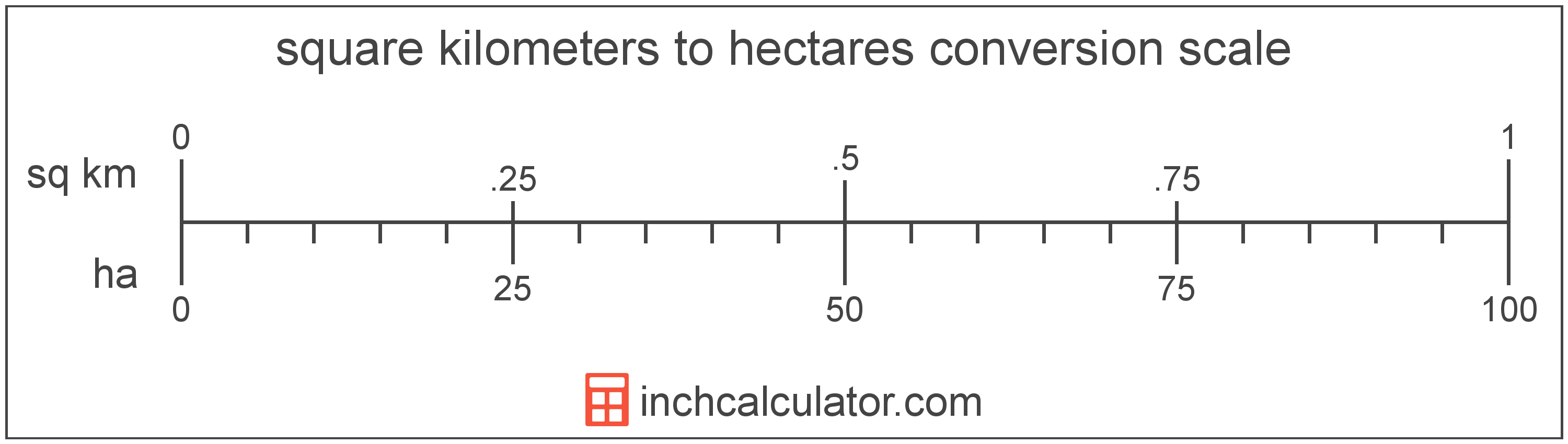# Square Kilometers to Hectares Converter

Enter the area in square kilometers below to get the value converted to hectares.

Results in Hectares:1 sq km = 100 ha

Do you want to convert hectares to square kilometers?

## How to Convert Square Kilometers to Hectares

To convert a measurement in square kilometers to a measurement in hectares, multiply the area by the following conversion ratio: 100 hectares/square kilometer.

Since one square kilometer is equal to 100 hectares, you can use this simple formula to convert:

hectares = square kilometers × 100

The area in hectares is equal to the area in square kilometers multiplied by 100.

For example, here's how to convert 5 square kilometers to hectares using the formula above.
hectares = (5 sq km × 100) = 500 ha### How Many Hectares Are in a Square Kilometer?

There are 100 hectares in a square kilometer, which is why we use this value in the formula above.

1 sq km = 100 ha

## What is a Square Kilometer?

One square kilometer is equivalent to the area of a square with sides that are each 1 kilometer in length. One square kilometer is equal to 0.386102 square miles or 1,000,000 square meters.

The square kilometer, or square kilometre, is a multiple of the square meter, which is the SI derived unit for area. In the metric system, "kilo" is the prefix for thousands, or 103. A square kilometer is sometimes also referred to as a square km. Square kilometers can be abbreviated as sq km, and are also sometimes abbreviated as km². For example, 1 square kilometer can be written as 1 sq km or 1 km².

## What is a Hectare?

One hectare is equal to 10,000 square meters, or the area of a square with 100 meter sides.

The hectare is an SI accepted unit for area for use with the metric system. In the metric system, "hecto" is the prefix for 102. Hectares can be abbreviated as ha; for example, 1 hectare can be written as 1 ha.

## Square Kilometer to Hectare Conversion Table

Table showing various square kilometer measurements converted to hectares.
Square Kilometers Hectares
1 sq km 100 ha
2 sq km 200 ha
3 sq km 300 ha
4 sq km 400 ha
5 sq km 500 ha
6 sq km 600 ha
7 sq km 700 ha
8 sq km 800 ha
9 sq km 900 ha
10 sq km 1,000 ha
11 sq km 1,100 ha
12 sq km 1,200 ha
13 sq km 1,300 ha
14 sq km 1,400 ha
15 sq km 1,500 ha
16 sq km 1,600 ha
17 sq km 1,700 ha
18 sq km 1,800 ha
19 sq km 1,900 ha
20 sq km 2,000 ha
21 sq km 2,100 ha
22 sq km 2,200 ha
23 sq km 2,300 ha
24 sq km 2,400 ha
25 sq km 2,500 ha
26 sq km 2,600 ha
27 sq km 2,700 ha
28 sq km 2,800 ha
29 sq km 2,900 ha
30 sq km 3,000 ha
31 sq km 3,100 ha
32 sq km 3,200 ha
33 sq km 3,300 ha
34 sq km 3,400 ha
35 sq km 3,500 ha
36 sq km 3,600 ha
37 sq km 3,700 ha
38 sq km 3,800 ha
39 sq km 3,900 ha
40 sq km 4,000 ha

## References

1. Cambridge Dictionary, hectare, https://dictionary.cambridge.org/us/dictionary/english/hectare